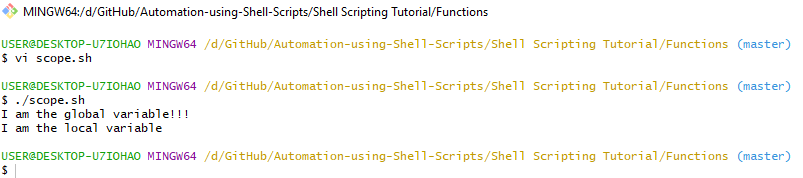×

Search anything:

# Functions in Shell Scripting

#### Software Engineering Shell ScriptingReading time: 30 minutes | Coding time: 10 minutes

In this article, we will go through how to use functions in shell scripting. We will cover the following sub-topics:

1. Creating a function in Shell
2. Passing parameters to function on call
3. Returning values from function
4. Nested function call
5. Scope of variables

Functions are subsets of a code that implement an independent programming logic.
For example, to create a four function calculator, independent programming logic are:

2. Multiplication function
3. Division function
4. Subtraction function

Function allows us to divide a larger problem into smaller independent problem which may lead to better understandability and debugging.

One major advantage of functions are code reuse.
A function, once written, need not to be defined again for doing a same task.
A task can be done in repetition easily with a function call using the function name.

### 1. Creating a function in Shell

To create a basic function in Shell Scripting, following syntax is followed:

Syntax:

```#Define function
{function name}()
{
Statements...
}
# Call the function
{function name}```
• Function name: It is a unique identifier for the function and its naming convention is similar to variables in Shell Scripting, i.e., A function name starts with _ or (a-z) or (A-Z) and may include digits. A function name cannot be a keyword defined in bash.
• Function call: A function in Shell is called by specifying its name.

Code:

```#!/bin/bash
# Create function
function1()
{
echo "Inside Function body";
}
# Invoke Function using function name
function1```

Here, a simple function is created, which when called, prints a message "Inside Function body" to the standard output.

Code in vi-editor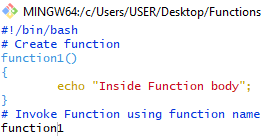Output: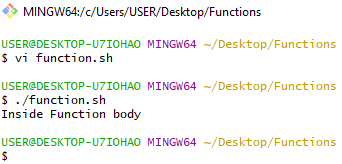### 2. Passing parameters to function on call

Parameters may be passed to function in Shell for further operations in a function.

Extending our analogy on the calculator example, we may pass parameter 2 and 4 to get addition output as 6 and on another function call we may pass parameter 3 and 5 to get addition output as 8.

Parameters in Shell are passed using the following syntax:

Syntax:

```#Define the function
{function name}()
{
# Access parameters using
# \$0 for file name (default)
# \$k for kth parameter
Statements...
}
# call the function by passing parameters
{function name} {parameter1} {parameter2} ...```

Here,

• \$0: defines the file name and it is passed by default.
• \$k: defines the kth parameter.

Code:

```#!/bin/bash
function1()
{
echo "Parameter 0 (File Name): \$0"
echo "Parameter 1: \$1"
echo "Parameter 2: \$2"
}
function1 p1 p2;```

Code in vi-editor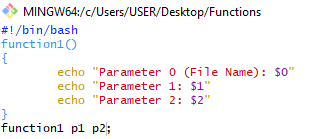Output: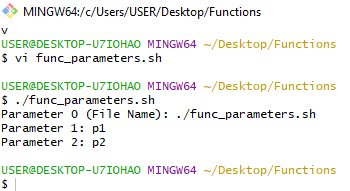Here, two parameters are passed namely "p1" and "p2" which are printed to the standard output.

### 3. Returning values from function

A function may return a value after doing some execution.
This value is returned to the calling environment (where function call is made) using the return keyword.

Note:

1. A function can return only one value.
2. Function value can be access using \$? in the calling environment.

Syntax:

```{function name}()
{
Statements...
return (value/variable);
}
# Call the function
{function name}
# Fetch the return variable using \$?
{variable}=\$?;```

Code:

```#!/bin/bash
function1()
{
return \$((\$1*\$2))
}
echo "Multiplication in Shell using Functions"
echo "Enter two numbers"
function1 \$num1 \$num2
mul=\$?
echo "Multiplied value is \$mul"```

Here,

• We read two numbers from the user from standard input.
• We call the function1 with both numbers as parameters.
• The function multiplies the parameters and returns the multiplied value.
• The returned value is assigned to the variable mul and printed to standard output with a message in the calling environment.

Code in vi-editor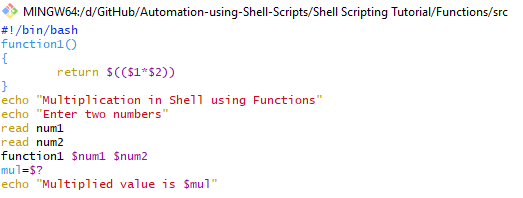Output: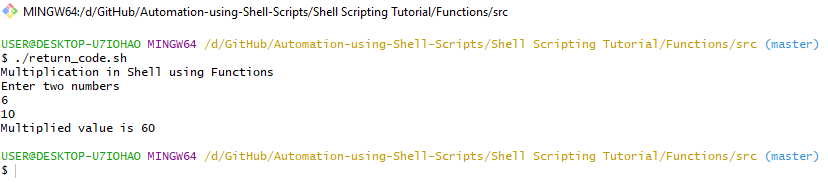Here, we pass two parameters 6 and 10 and function returns a multiplied value: 6 x 10 = 60.

### Fetching returned value when multiple functions are called

Following are the basics of functions in Shell:

• A fruitful function (that returns a value) can return only one value at a time.
• The returned value can be accessed only by using \$? symbol.

Thus, if multiple functions are called, the \$? symbol keeps the returned value of only the last called functions because all previous values are overwritten when another function is called.

Case 1: Multiple functions are called and output of returned value is printed at last

Code

```#!/bin/bash
{
return \$((\$1+\$2))
}
multiply()
{
return \$((\$1*\$2))
}
# Call Addition for 3 and 4 == 7
# Call multiplication for 3 and 4 == 12
multiply 3 4
# Call Addition for 5 and 4 == 9
# Store answer (always stores final function call returned value)
ans=\$?
echo "\$ans"```

Code in vi-editor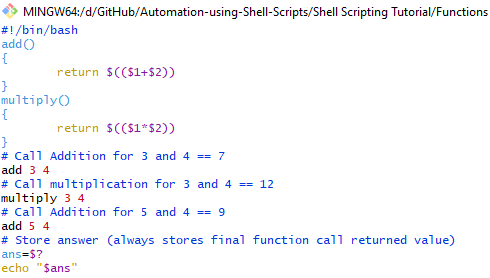Here, multiple functions are called simultaneously without printing the return value of each function call.
At last, \$? fetches return value of last function call and prints it to the standard output.

Output: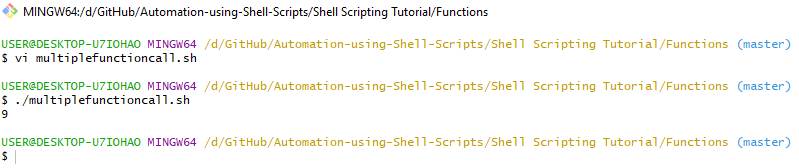Here, the \$? only accessed the return value of the last called function which performs addition of 5 and 4 to give output as 9.

Case 2: Multiple functions are called and output of returned value is printed along with function call

Code:

```#!/bin/bash
{
return \$((\$1+\$2))
}
multiply()
{
return \$((\$1*\$2))
}

# Call Addition for 3 and 4 == 7
echo "\$?"

# Call multiplication for 3 and 4 == 12
multiply 3 4
echo "\$?"

# Call Addition for 5 and 4 == 9
echo "\$?"```

Code in vi-editor: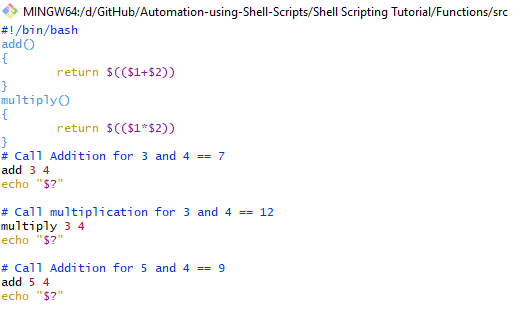Here, multiple functions are called and their returned values are printed simultaneously to the standard output.

Output: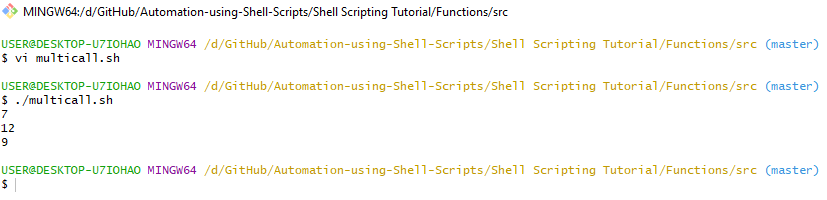Here, three functions are called and their output are printed at separate lines:

1. Addition of 3 and 4: 7
2. Multiplication of 3 and 4: 12
3. Addition of 5 and 4: 9

### 4. Nested function call

If a function calls another function, is leads to nesting of functions.

Note: A function may call any function irrespective of whether it is defined above it in the source code or below because functions are static code.

Syntax:

```# Define a function
{fun1}()
{
Statements...
# Call another function
{fun2}
}
{fun2}()
{
Statements...
}
# Call either function that is calling the other function
{fun1}```

Code:

```#!/bin/bash
echo "Nested functions"
# First function
function1()
{
echo "Function 1 body"
function2;
}
# Second function
function2()
{
echo "Function 2 body"
}
function1```

Here,

• Function1 is called by the calling environment and prints the message "Function1 body"
• Function 1 calls the Function2 and prints the message "Function2 body"
• Function 2 returns the control to the function 1.
• Since no other statement is defined after the function call, function 1 returns the control to the main calling environment.

Code in vi-editor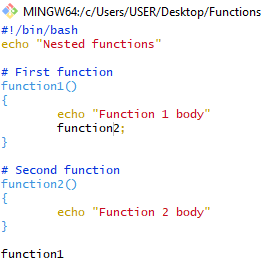Output: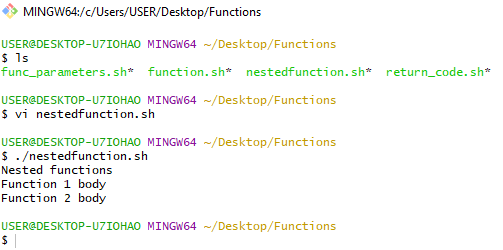### 5. Scope of variables

Scope or lifetime of a variable defines the code block in which a variable exists and can be worked upon.

Based on scope, variables are of two types:

1. Local variables: Local variables are defined in a code block { }.
Example: Variables declared in function.
Their use is limited within the code block and cannot be used outside their scope.

2. Global variable: Global variables, generally defined at the top of Shell code after invoking the bash, have scope of the entire source code, i.e, they can be used and accessed anywhere in the entire code.

Code:

```#!/bin/bash
# Declaring a global variable
gvar="I am the global variable!!!"

# Define a function
function1()
{
# Declaring a local variable
lvar="I am the local variable"
echo "\$gvar"
echo "\$lvar"
}

# Call the function
function1```

Code in vi-editor: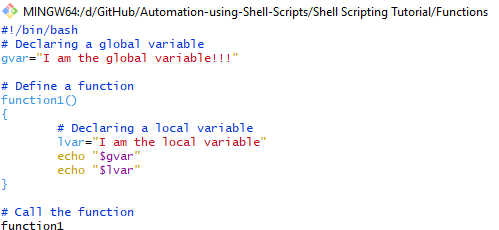Here,

• A global variable gvar is declared at the top.
• a local variable lvar is declared within a function function1
• Both the variables are printed inside the function body.

Output: